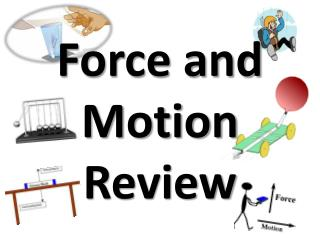DownloadDownload PresentationForce and Motion Review

# Force and Motion Review

Download Presentation## Force and Motion Review

- - - - - - - - - - - - - - - - - - - - - - - - - - - E N D - - - - - - - - - - - - - - - - - - - - - - - - - - -

1. ### Force and Motion Review

2. A force is simply a pushor a pull. All forces have both size and direction.
3. Do you remember… BrainPop: Force video clip
4. NET FORCES When two or more forces are combined!
5. Some tips: Forces in the same direction- add the two forces together. + = Forces in different directions-subtract the two and figure out which direction was the stronger of the two. - =
6. Balanced vs. unbalanced forces Unbalanced: when the net force on an object is not zero. These produce a change in motion. Balanced: when the net force on an object equals zero. These do NOT produce change in motion.
7. What is motion? When an object changes position over time when compared with a reference point we say that the object is in motion.
8. Speed The rate at which an object moves. Depends on distance traveled and the time it takes to travel that distance.
9. Speed Formula Average speed = total distance total time
10. Velocity The speed of an object in a particular direction ***Don’t confuse this with speed…they don’t mean the same thing!!
11. Velocity must ALWAYS include a direction Ex: an airplane’s velocity might be 600 km/h south
12. Velocity changes as speedORdirection changes
13. Acceleration The rate at which velocity changes. To change velocity (change speed or direction or BOTH) BrainPop: Acceleration video clip
14. Acceleration Remember acceleration involves three things: Speeding up (this will be positive) Slowing down (this will be negative) Turning or changing direction
15. It is not just HOW MUCH velocity changes, but it is alsoHOW FAST it changes. The faster velocity changes, the greater the acceleration is.
16. Acceleration Formula Acceleration= final velocity – starting velocity time it takes to change velocity Velocity is expressed in meters per second (m/s) and time is expressed in seconds (s), so acceleration is expressed in meters per second per second (m/s/s)
17. 1 3

### Newton’s Laws of Motion

2 BrainPop: Newton’s Laws of Motion video clip
18. Newton’s First Law(law of inertia) 1 An object at rest tends to stay at rest and an object in motion tends to stay in motion unless acted upon by an unbalanced force.
19. Balanced Force Equal forces in opposite directions produce no motion
20. Unbalanced Forces Unequal opposing forces produce an unbalanced force causing motion
21. Newton’s First Law (law of inertia) Mass (kg)is the measure of the amount of matter in an object. INERTIA is a property of an object that describes how much it will resist change to the motion of the object More mass an object has means more inertia the object will have.
22. What is this unbalanced force that acts on an object in motion? Friction! There are fourmain types of friction: Sliding friction: ex. ice skating Rolling friction: ex. bowling Fluid friction (air or liquid): ex. air or water resistance Static friction: ex. initial friction when moving an object
23. Friction! ADVANTAGES Helps us to move (example: walking) Helps us do work (example: writing) Helps us get from place to place (example: driving car on road)
24. Friction! DISADVANTAGES Makes movement difficult (remember your rusty bike chain, it makes pedaling difficult) Wastes energy (when friction is high, it takes more energy to move an object) Heats things up (too much heat can cause fire) Wears things out (look at the bottom of your shoes)
25. Friction! You can reduce friction by adding lubricant to an object. Think about adding water to your slide, oil on your bike chain, wax to your car, furniture polish to the hardwood floors, ice on the pavement. How could you increase friction?
26. This will help up remember
27. Newton’s Second Law 2 Force equals mass times acceleration.
28. Newton’s Second Law Force = Mass x Acceleration Force is measured in Newtons ACCELERATION of GRAVITY Earth) = 9.8 m/s2 WEIGHT is a measure of the force of gravity on the mass of an object Weight (force) = mass x gravity (Earth) BrainPop: Gravity video clip
29. This will help up remember: Newton’s Second Law video clip
30. Who would you choose to be your scooter board partner? How does this affect acceleration? If you weighed 70 pounds and your scooter board partner weighed 120, how would this affect your acceleration? What about momentum? What would happen if the you switched positions?
31. Newton’s Third Law 3 For every action there is an equal and opposite reaction.
32. Newton’s 3rd Law For every action there is an equal and opposite reaction. Book to earth Table to book
33. Think about it . . . What happens if you are standing on a skateboard or a slippery floor and push against a wall? You slide in the opposite direction (away from the wall), because you pushed on the wall but the wall pushed back on you with equal and opposite force. Why does it hurt so much when you stub your toe? When your toe exerts a force on a rock, the rock exerts an equal force back on your toe. The harder you hit your toe against it, the more force the rock exerts back on your toe (and the more your toe hurts).
34. Action and Reaction on Different Masses Consider you and the earth Action: earth pulls on you Reaction: you pull on earth